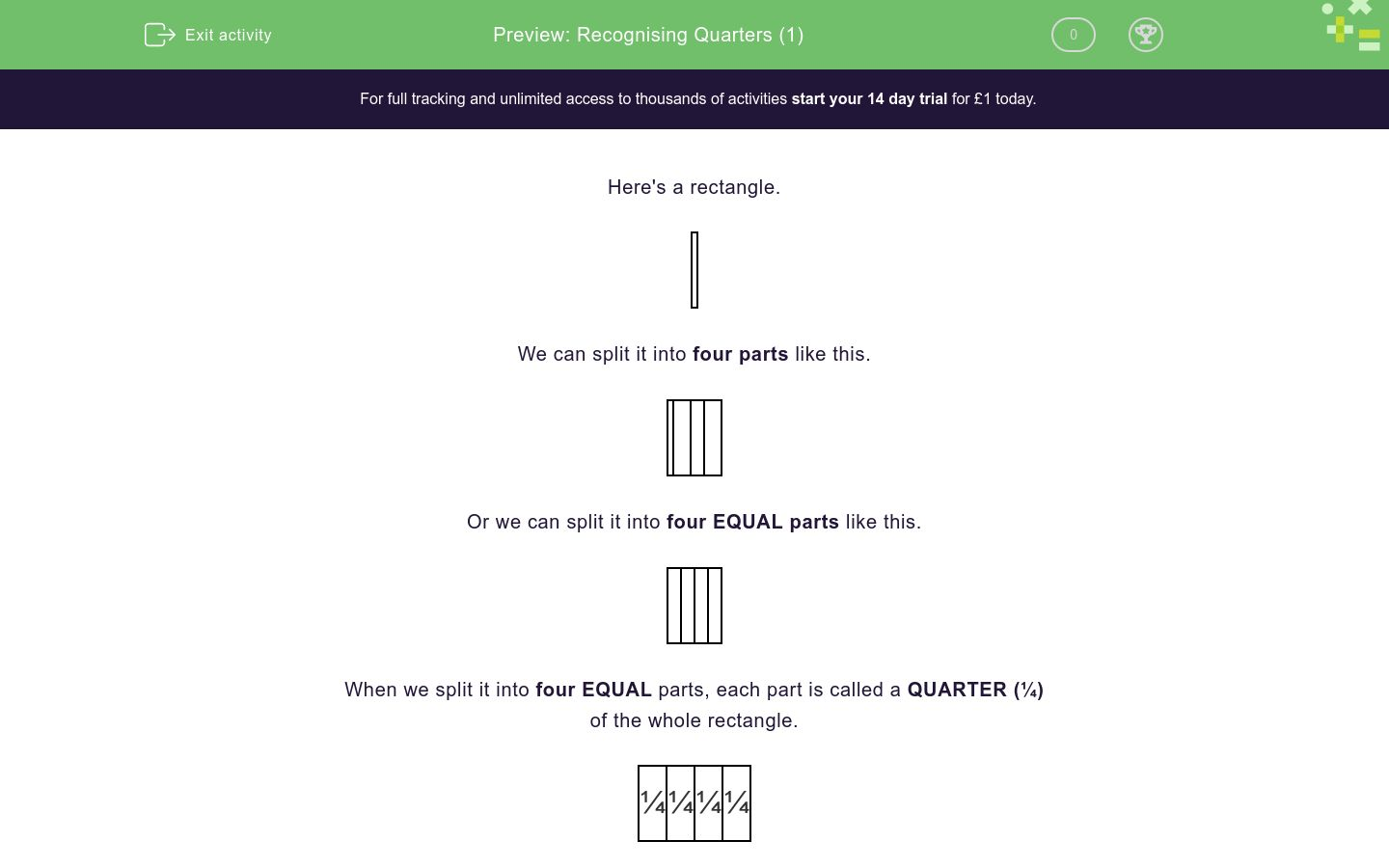# Recognising Quarters (1)

In this worksheet, students must recognise quarters as one of four equal parts in a shape.Key stage:  KS 1

Curriculum topic:  Number: Fractions

Curriculum subtopic:  Recognise Halves and Quarters

Difficulty level:### QUESTION 1 of 10

Here's a rectangle.

We can split it into four parts like this.

Or we can split it into four EQUAL parts like this.

When we split it into four EQUAL parts, each part is called a QUARTER (¼)

of the whole rectangle.

 ¼ ¼ ¼ ¼

When the parts are not EQUAL, they are not quarters.

Is this true or false?

This shape shows QUARTERS.

true

false

Is this true or false?

This shape shows QUARTERS.

true

false

Is this true or false?

This shape shows QUARTERS.

true

false

Is this true or false?

This shape shows QUARTERS.

true

false

Is this true or false?

This shape shows QUARTERS.

true

false

Is this true or false?

This shape shows QUARTERS.

true

false

Is this true or false?

This shape shows QUARTERS.

true

false

Is this true or false?

This shape shows QUARTERS.

true

false

Is this true or false?

This shape shows QUARTERS.

true

false

Is this true or false?

This shape shows QUARTERS.

true

false

• Question 1

Is this true or false?

This shape shows QUARTERS.

CORRECT ANSWER
false
EDDIE SAYS
The parts are not equal and there are only two of them, not four.
• Question 2

Is this true or false?

This shape shows QUARTERS.

CORRECT ANSWER
false
EDDIE SAYS
The parts are not equal.
• Question 3

Is this true or false?

This shape shows QUARTERS.

CORRECT ANSWER
true
EDDIE SAYS
The four parts are equal.
• Question 4

Is this true or false?

This shape shows QUARTERS.

CORRECT ANSWER
true
EDDIE SAYS
The four parts are equal.
• Question 5

Is this true or false?

This shape shows QUARTERS.

CORRECT ANSWER
false
EDDIE SAYS
The parts are not equal.
• Question 6

Is this true or false?

This shape shows QUARTERS.

CORRECT ANSWER
false
EDDIE SAYS
There are three parts, not four.
• Question 7

Is this true or false?

This shape shows QUARTERS.

CORRECT ANSWER
true
EDDIE SAYS
The four parts are equal.
• Question 8

Is this true or false?

This shape shows QUARTERS.

CORRECT ANSWER
false
EDDIE SAYS
The parts are not equal.
• Question 9

Is this true or false?

This shape shows QUARTERS.

CORRECT ANSWER
true
EDDIE SAYS
The four parts are equal.
• Question 10

Is this true or false?

This shape shows QUARTERS.

CORRECT ANSWER
true
EDDIE SAYS
The four parts are equal.
---- OR ----

Sign up for a £1 trial so you can track and measure your child's progress on this activity.

### What is EdPlace?

We're your National Curriculum aligned online education content provider helping each child succeed in English, maths and science from year 1 to GCSE. With an EdPlace account you’ll be able to track and measure progress, helping each child achieve their best. We build confidence and attainment by personalising each child’s learning at a level that suits them.

Start your £1 trial